# A CMOS Pre-Amp for Hi-Z sources

A few posts back we took a look at the challenges of measuring high-Z sources with audio analyzers than have a bi-polar based front-end. The big trade off, of course, is that BJT pre-amps have great noise with lower impedance sources, and CMOS have great noise with very high impedance sources. Where this cross-over occurs depends. But it can be in the 1 to 10 KHz range--lower than you might expect.

For this post, we built a simple battery-powered CMOS pre-amp with the goal of measuring the thermal noise in a 500K ohm resistor. The schematic of this circuit is shown below:The circuit is very simple: On the left is a 2032 coin cell. There are two resistors that split the coin cell voltage in half, and an OPA377 is used to buffer the resistor voltage. That signal is then fed into the QA401.

The aim here was to measure the noise density at 1 KHz, and set the system up so that the noise at 1 KHz was overwhelmingly from the resistors. There are a few challenges to keep in mind. The first is that the opamp, being CMOS, will have fairly high levels of 1/f voltage (aka flicker) noise. This is noise that exhibits a 1/f rolloff, and there's a good chance it can swamp out the signal of interest if that interest is too low in frequency.

The second is the input capacitance of the opamp. With such large resistors on the input, the stray C of the input becomes important. The R and C could combine and suckout the noise before it could be measured.

Thus, our goal is to measure the noise of a resistor, in a region above the 1/f noise, and also below where LPF formed with the stray opamp input capacitance. Since the capacitance issue is easier to figure out, let's start there.

Below is the input capacitance of the OPA377, taken from the spec sheet: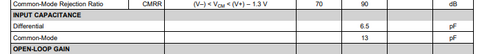If we assume the layout contributes another 10-15 pF, then we might be looking at a total input capacitance of 20 to 30 pF. Let's pick 30 pF. The 30 pF will form a corner with the R equivalent (1M || 1M = 500K) around 10 KHz. Which means for measuring noise at 1 KHz, we probably don't need to worry about the that.

The opamp's input voltage noise plot is shown below:A 500K ohm resistor will have a noise density around 90nV/rthz, and so we can see the opamp voltage noise doesn't factor in at all here, mostly because we're at a gain of 1. In fact, we could drop down to 50K and still be dominated by the resistor noise.

For current input noise, TI indicates a current noise density of 2 fA/rthz at 1 KHz. The graph of current noise density has a 1/f region too. In the data sheet, where the knee occurs isn't mentioned. But since TI has specified the current noise at 1 KHz, we can assume that is beyond the knee and into the flat white noise region.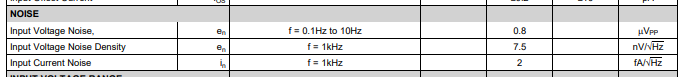In the end, if you run the numbers, the output noise at the amplifier at 1 KHz output is solely determined by the resistor noise. And that is by design. Remember, the goal was to isolate the resistor so that we could measure its noise exclusively. And so far, the simple circuit has done that.

## What is the Noise Index?

The math above is pretty straightforward, and you can find a good treatment of the processes to arrive at these numbers in numerous app notes and on-line discussions.

And while it's easy to find the theoretical thermal noise in a resistor, there is another phenomenon that is less widely know or understood by engineers: Current noise. Current noise is a noise that is proportional to the square of the DC voltage drop across the resistor. It's also inversely proportional to the frequency.

Vishay has a great app note that discusses this in more detail here, with the salient graph from that note shown below: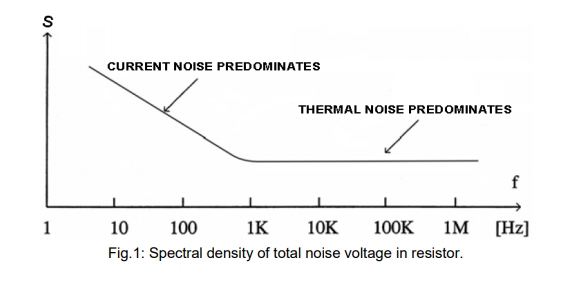Remember, if there is 0 volts across the resistor, then the current noise is also zero. In the case of this circuit, we have 3V across a 2M resistor. That puts our corner frequency around 120 Hz given the thin-film 0603 resistors we used in the proto, which means the noise at 1K is dominated by the thermal noise.

Something that matters a lot is the noise index of the resistors used. Above I noted Thin Film was used. Was it really needed? The answer to that is "probably". And to be even more certain, large 0805 thin films should be used for even more margin.

Below is a plot from the Vishay note, highlighting the noise index of common resistor formulations.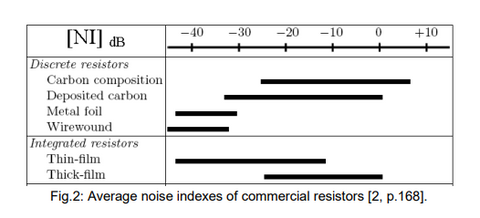The noise index is specified in dB, which can be confusing. dB of what? The noise index is the noise (in microvolts) per volt of bias per decade of frequency. So, if you see a Noise Index of -10 dB, then this means you have 0.316 uV / volt / decade.

A 10K ohm resistor with a 0 dB noise index biased at 10V would give you 1 uV * 10 = 10uV of noise per decade of frequency. From 20 to 20 KHz, that would be 17.3 uV total noise current (the multiplier is sqrt(ln(f2/f1)/2.3), which for f2=20K and f1 = 20, you get 1.73, so 1uV * 10V bias * 1.73 bandwidth adjustment).

That 10K resistor, from 20 to 20 KHz, would also generate 1.8uV of total voltage noise. Taking the square of each, adding, followed by the square root would give total resistor noise. But it's 99% dominated by the current noise.

Now, that was using a thick film resistor at the high end of the scale (0 dB noise index). If we switch to a thin film, it gets better. But what determines where you sit in the ranges displayed because he ranges in the graph above are huge: 30 dB or more! In other words, what decisions cause you to end up on the right side of the scale (worse) versus left side of the scale (better)? The biggest contributor is resistor physical size (bigger is better), followed by resistor value (lower is better). So, if we go with a 0402 thick film with a 0 dB noise index, our 10K resistor biased at 10V is overwhelmingly current noise.

But if we go with a 1206 thin film part with a -40 dB noise index, then we're back to being dominated by voltage noise.

And ultimately, that's what we want in this experiment: To be dominated by resistor thermal voltage noise. A 1Mohm 0603 thin film resistor with a noise index of 0 dB, biased at 3V gives you a corner frequency just over 100 Hz. And thus our goal of measuring purely the resistor voltage noise density at 1 KHz is maintained.

The Vishay series used for this board is here, and the plot of the current noise voltage ratio is shown below.Recall that 1 uV/V is a noise index of 0 dB. And as you can see, the 0603 at 1M hits the 0 dB mark precisely.

The summary is that given the size and value of the resistor, the crossover should be a few hundred Hz. Below that, and 1/f noise dominates. Above that, and thermal noise dominates. Because our goal is to measure resistor thermal noise at 1 KHz, this crossover is good.

## The Measurements

OK, so what do we measure?

First, let's look at the QA401 with inputs shorted and the attenuator engaged. This effectively measures the 10K input shunt resistor that forms the attenuator. This is coming in around -125 dBV/rthz, while the 10K resistor should be -158 dBV/rthz. And remember from the blog post linked at the very top of this post, that is due to the bias current noise of the OPA1612. This is roughly a 30 dB degradation due to opamp bias current!Next, this is the output of the CMOS amp we built: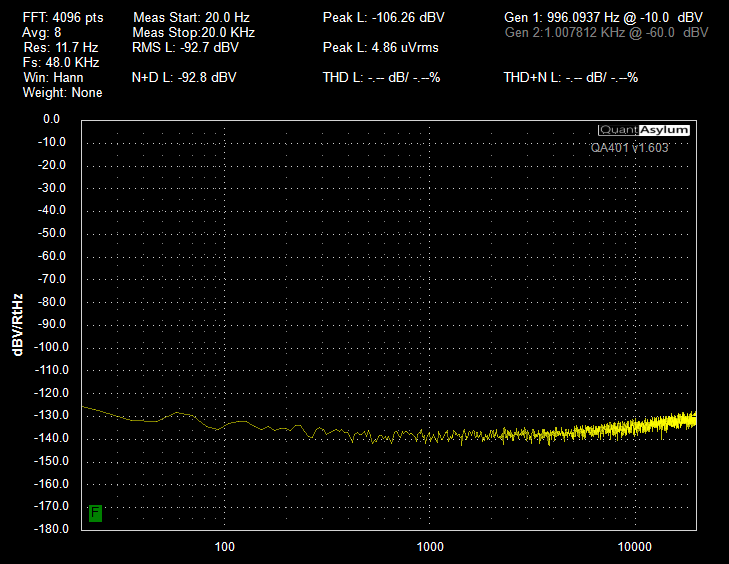The thermal noise of a 500K resistor (the parallel equivalent of the two 1M parts used in the circuit) is -140.8 dBV/rthz. Above, we measured about -139 dBV/rthz. Very good agreement.

The very simple CMOS pre-amp shows a 15 dB improvement with impedances in the 100K+ ohm range. Of course, the CMOS pre-amp's noise voltages would be a disaster for measuring lower impedance sources as the voltage noise is much to high.

## Summary

The original desire was to use a high-valued resistor to confirm the math used in the QA401 for computing noise density. With a CMOS pre-amp and some carefully chosen component values, that math has been verified for a 500K equivalent resistor, further building confidence in the latest software release.

At some point in the future, we'll extend this test setup down to 50Kohm resistor values, and also take a look at measuring the noise index of these resistors.